# Kmeans++聚类算法原理与实现

## 1 引例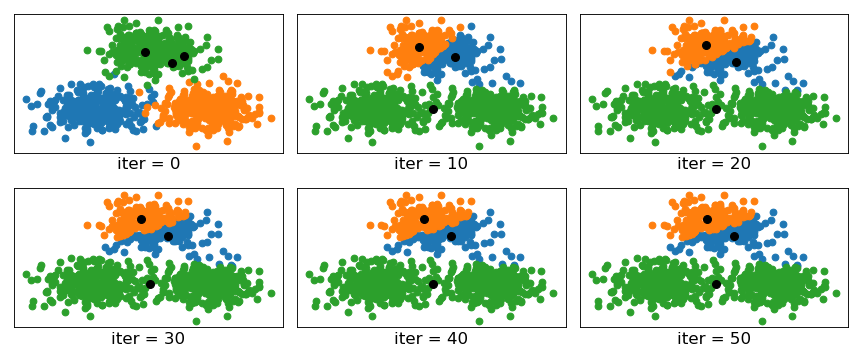## 2 Kmeans++聚类算法

K m e a n s + + Kmeans++ ，仅从名字也可以看出它就是 K m e a n s Kmeans 聚类算法的改进版，那它又在哪些地方对 K m e a n s Kmeans 进行了改进呢？一言以蔽之， K m e a n s + + Kmeans++ 算法仅仅只是在初始化簇中心的方式上做了改进，其它地方同 K m e a n s Kmeans 聚类算法一样。

### 2.1 Kmeans++原理

K m e a n s + + Kmeans++ 在初始化簇中心时的方法总结成一句话就是：**逐个选取 k k 个簇中心，且离其它簇中心越远的样本点越有可能被选为下一个簇中心。**其具体做法如下（其中引用英文部分论文原文）：

①从数据集 X \mathcal{X} 中随机（均匀分布）选取一个样本点作为第一个初始聚类中心 c i c_i ;

1a. Take one center c 1 c_1 , chosen uniformly at random from X \mathcal{X} .

②接着计算每个样本与当前已有聚类中心之间的最短距离，用 D ( x ) D(x) 表示；然后计算每个样本点被选为下一个聚类中心的概率 P ( x ) P(x) ，最后选择最大概率值所对应的样本点作为下一个簇中心；

1b. Take a new center c i c_i , choosing x ∈ X x\in\mathcal{X} with probability P ( x ) P(x) .

P ( x ) = D ( x ) 2 ∑ x ∈ X D ( x ) 2 (1) P(x)=\frac{D(x)^2}{\sum_{x\in\mathcal{X}}D(x)^2}\tag{1}

③重复第②步，直到选择出 k k 个聚类中心；

1c. Repeat Step 1b. until we have taken k k centers altogether.

### 2.2 计算示例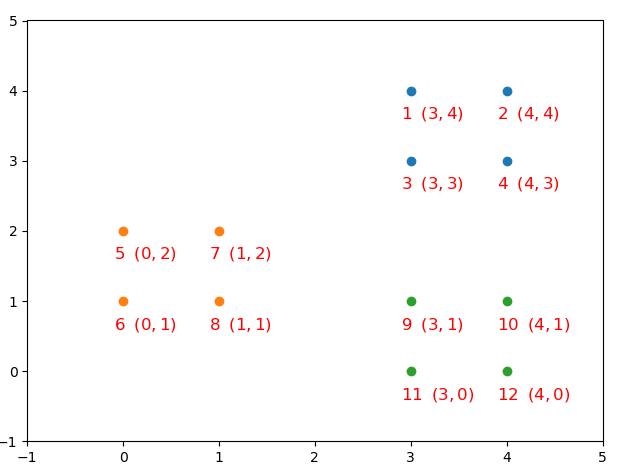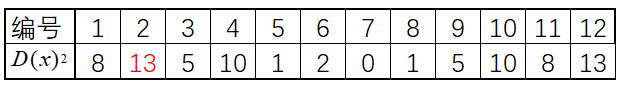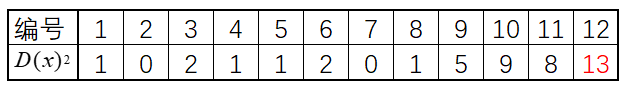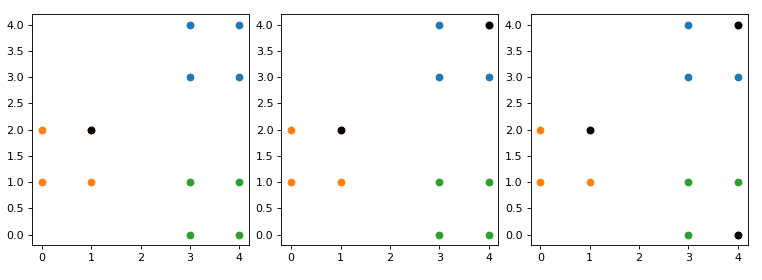### 2.3 编程实现

def InitialCentroid(x, K):
c0_idx = int(np.random.uniform(0, len(x)))
centroid = x[c0_idx].reshape(1, -1)  # 选择第一个簇中心
k = 1
n = x.shape
while k < K:
d2 = []
for i in range(n):
subs = centroid - x[i, :]
dimension2 = np.power(subs, 2)
dimension_s = np.sum(dimension2, axis=1)  # sum of each row
d2.append(np.min(dimension_s))
new_c_idx = np.argmax(d2)
centroid = np.vstack([centroid, x[new_c_idx]])
k += 1
return centroid

if __name__ == '__main__':
x, y = make_data()
K = len(np.unique(y))
# y_pred = kmeans(x, y)
y_pred = kmeanspp_visual(x,y,K)
nmi = normalized_mutual_info_score(y, y_pred)
print("NMI by ours: ", nmi)

model = KMeans(n_clusters=K, init='k-means++')
model.fit(x)
y_pred = model.predict(x)
nmi = normalized_mutual_info_score(y, y_pred)
print("NMI by sklearn: ", nmi)

#结果：
NMI by ours:  0.9456935014894648
NMI by sklearn:  0.9456935014894648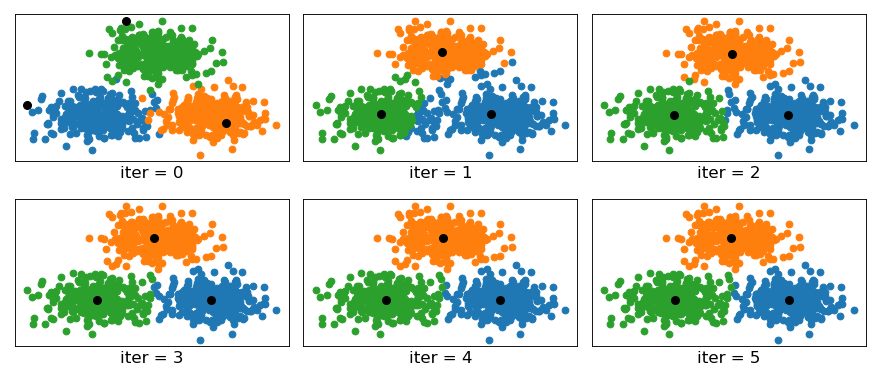## 3 总结

### 引用

Kmeans++ http://ilpubs.stanford.edu:8090/778/1/2006-13.pdf

示例代码 ： https://github.com/moon-hotel/MachineLearningWithMe

### 近期文章

Kmeans聚类算法

原来这就是支持向量机

朴素贝叶斯算法08-21161907-072348
05-168万+
07-08
02-289万+
03-105251
12-2521
08-083万+
03-11806
06-181万+
07-081万+
01-111081
12-031006
04-241万+
06-01735